#The Wolfram Language:Fast Introduction for Programmers

All sections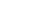Get Started

# Pure FunctionsVideo Version

The Wolfram Language allows what it calls pure functions, indicated by ending with &
Their first argument is indicated by `#`

(These are also known as anonymous functions, lambda expressions, etc.)

Notes for Java programmers:

Pure functions work similarly to lambda expressions in Java, but the syntax is simpler and more consistent in the Wolfram Language.

Notes for Python programmers:

Pure functions in the Wolfram Language work similarly to lambda functions in Python. The language.wlexpr function from the Wolfram Client Library for Python can be used to define and evaluate Wolfram Language pure functions from a Python environment.

Make a pure function for adding 1:

In:=1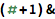`(# + 1) &`
Out=1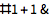If a pure function is given as the head of an expression, the function is applied to the arguments:

In:=2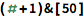`(# + 1) &`
Out=2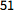Here is a function of several arguments:

In:=3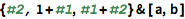`{#2, 1 + #1, #1 + #2} &[a, b]`
Out=3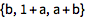This is an alternative way to specify the function:

In:=4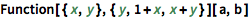`Function[{x, y}, {y, 1 + x, x + y}][a, b]`
Out=4Notes for Java programmers:

Wolfram Language pure functions provide a much simpler syntax for including multiple parameters than Java lambda expressions.

Notes for Python programmers:

Wolfram Language pure functions can indicate parameters either with # or by giving them explicit names. Python's lambda functions always require named parameters.

Lots of built-in functions commonly use pure functions:

In:=1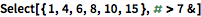`Select[{1, 4, 6, 8, 10, 15}, # > 7 &]`
Out=1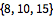In:=2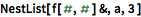`NestList[f[#, #] &, a, 3]`
Out=2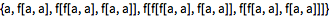Which of the following is a pure function that adds two numbers?

Which of these is the value of (`#` + 2) & ?

Which of these is the output of {`#`2, `#`1, `#`3} & [2, 3, 4, 5]?